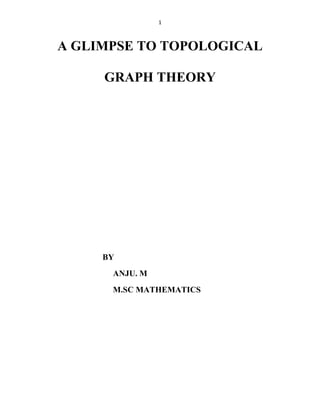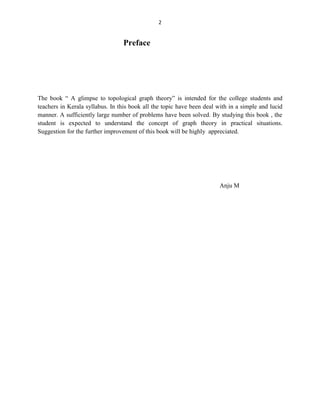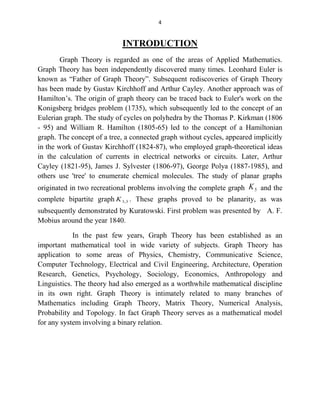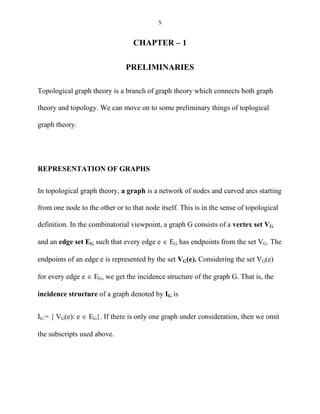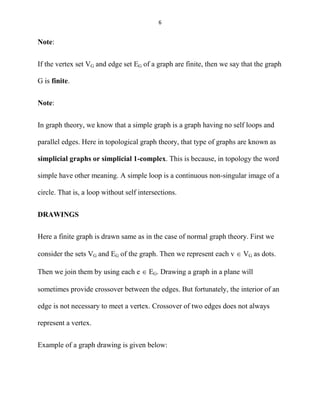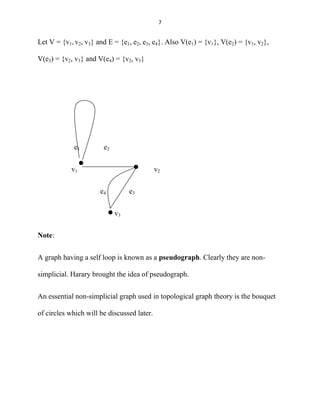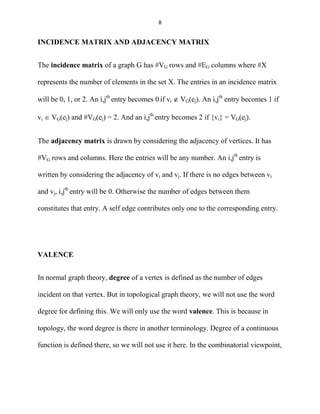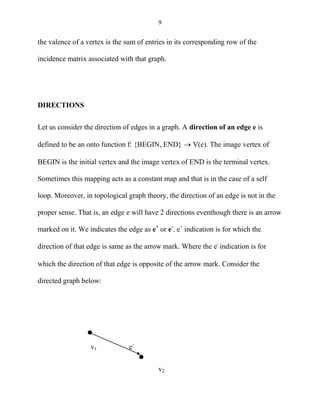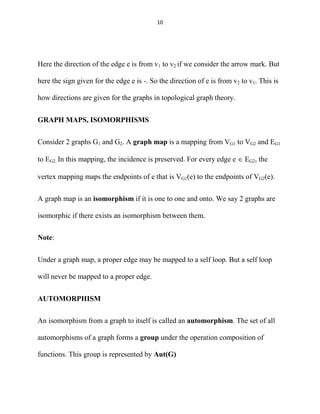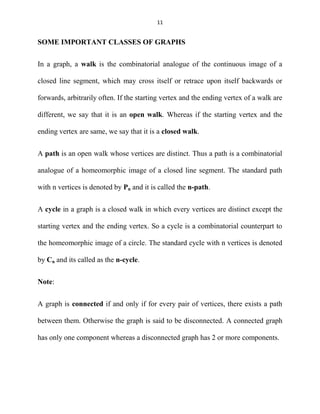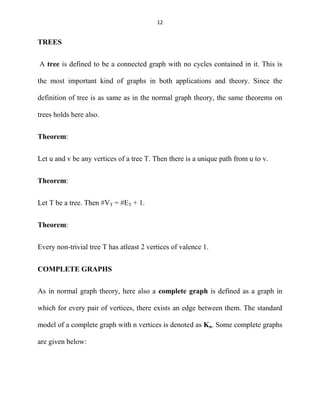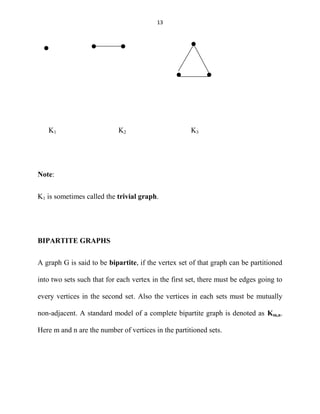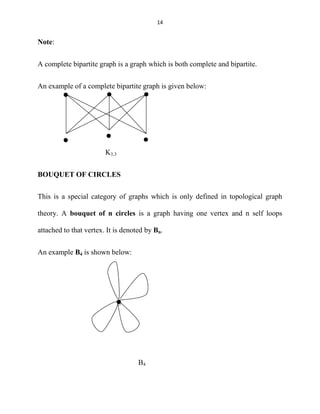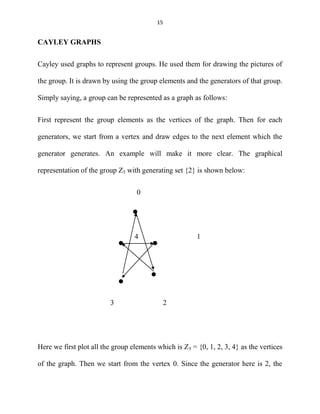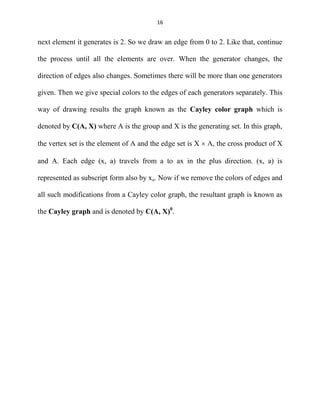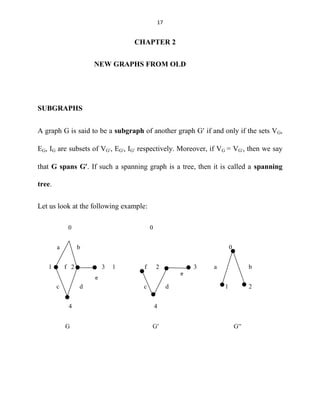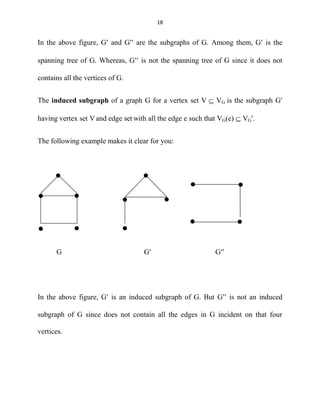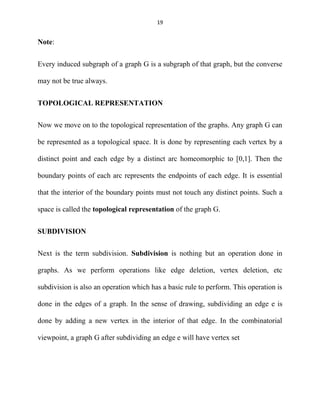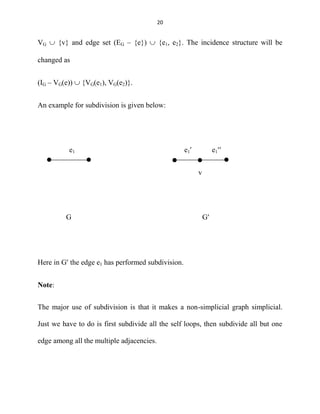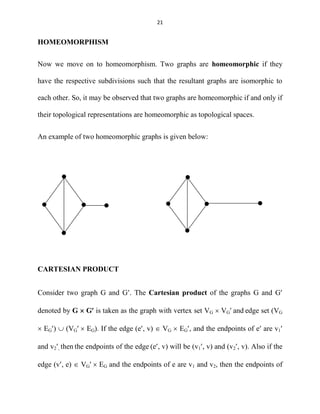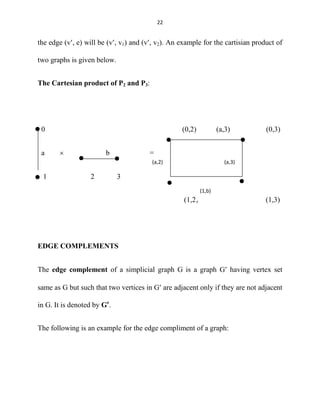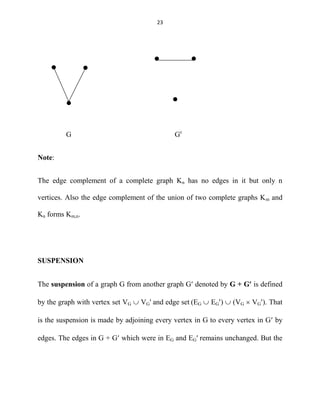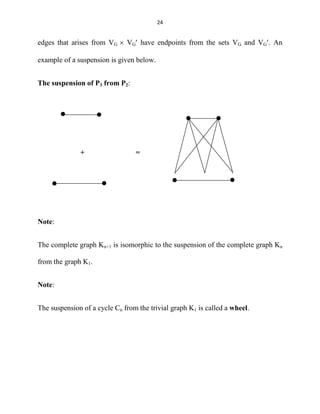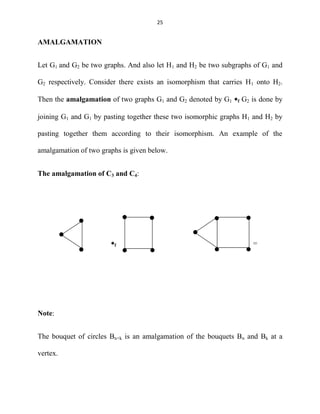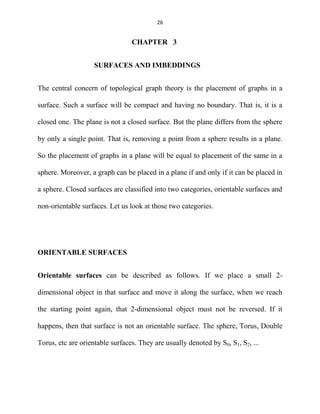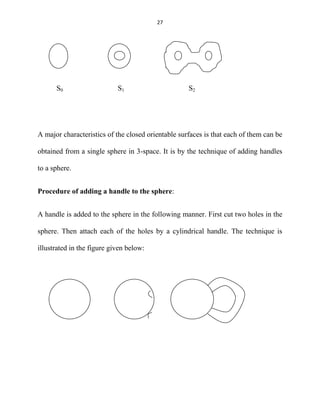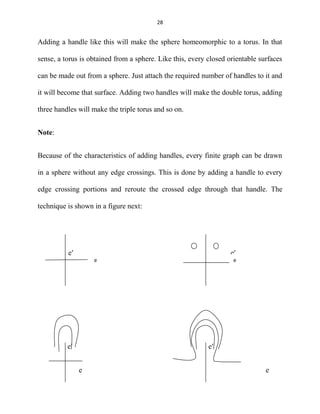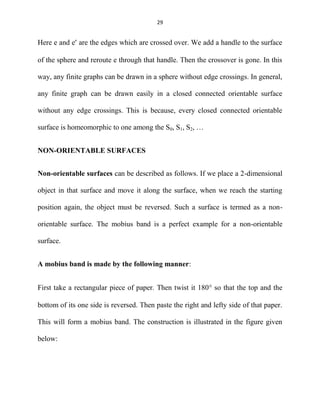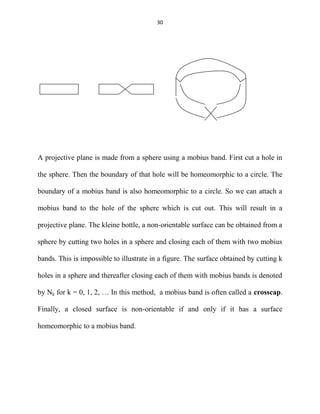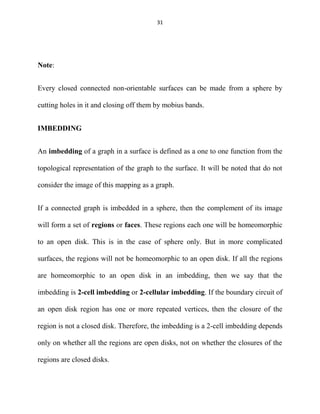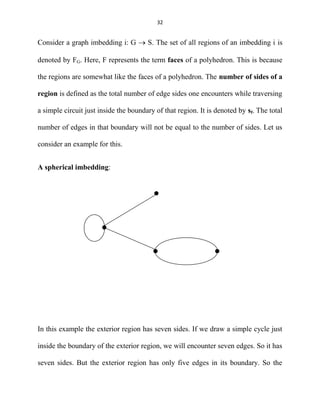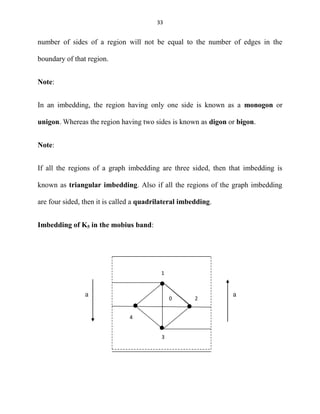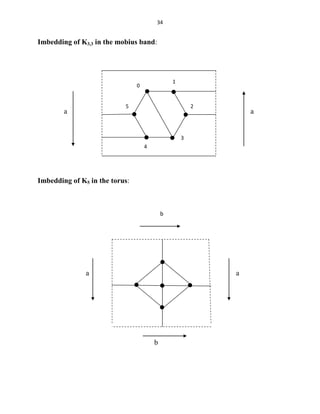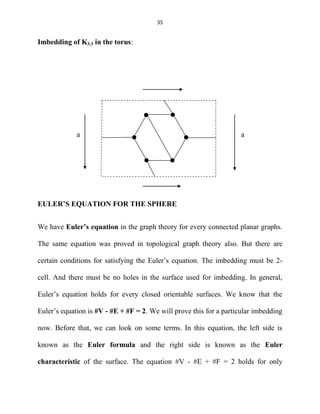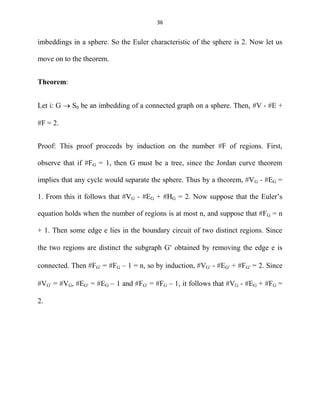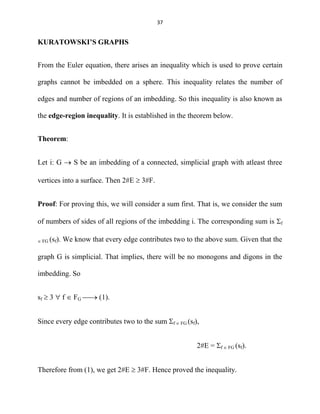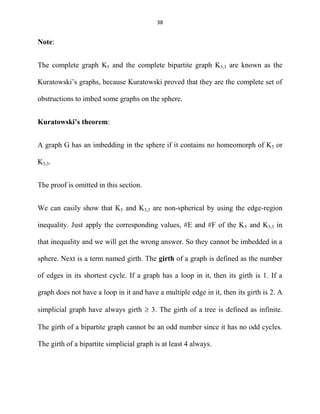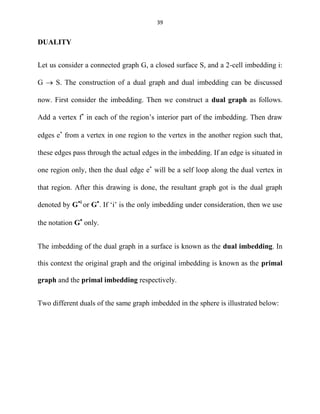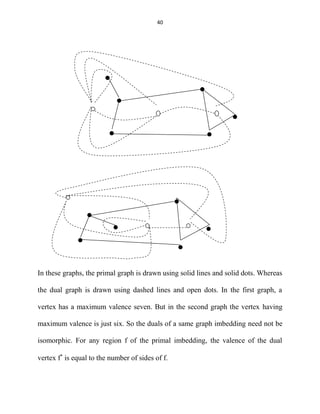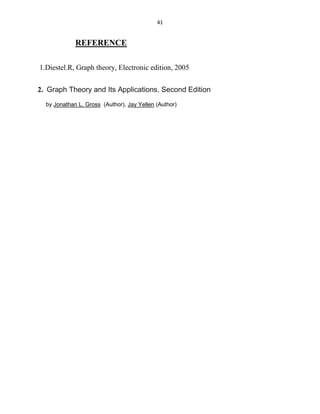Próximo SlideShare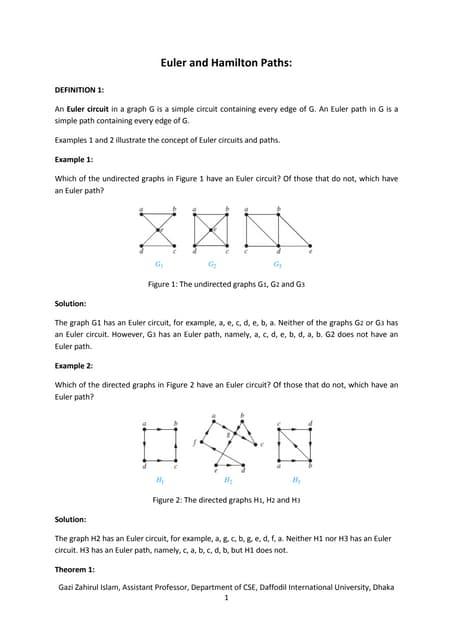Euler and hamilton paths
Cargando en ... 3
1 de 41

### A glimpse to topological graph theory

1. 1 A GLIMPSE TO TOPOLOGICAL GRAPH THEORY BY ANJU. M M.SC MATHEMATICS
2. 2 Preface The book “ A glimpse to topological graph theory” is intended for the college students and teachers in Kerala syllabus. In this book all the topic have been deal with in a simple and lucid manner. A sufficiently large number of problems have been solved. By studying this book , the student is expected to understand the concept of graph theory in practical situations. Suggestion for the further improvement of this book will be highly appreciated. Anju M
3. 3 CONTENTS Title Page No: Preface Chapter 1 Preliminaries 5 Chapter 2. New graphs from old 17 Chapter 3. Surfaces and imbedding 26 Reference 41
4. 4 INTRODUCTION Graph Theory is regarded as one of the areas of Applied Mathematics. Graph Theory has been independently discovered many times. Leonhard Euler is known as “Father of Graph Theory”. Subsequent rediscoveries of Graph Theory has been made by Gustav Kirchhoff and Arthur Cayley. Another approach was of Hamilton‟s. The origin of graph theory can be traced back to Euler's work on the Konigsberg bridges problem (1735), which subsequently led to the concept of an Eulerian graph. The study of cycles on polyhedra by the Thomas P. Kirkman (1806 - 95) and William R. Hamilton (1805-65) led to the concept of a Hamiltonian graph. The concept of a tree, a connected graph without cycles, appeared implicitly in the work of Gustav Kirchhoff (1824-87), who employed graph-theoretical ideas in the calculation of currents in electrical networks or circuits. Later, Arthur Cayley (1821-95), James J. Sylvester (1806-97), George Polya (1887-1985), and others use 'tree' to enumerate chemical molecules. The study of planar graphs originated in two recreational problems involving the complete graph 5K and the complete bipartite graph 3,3K . These graphs proved to be planarity, as was subsequently demonstrated by Kuratowski. First problem was presented by A. F. Mobius around the year 1840. In the past few years, Graph Theory has been established as an important mathematical tool in wide variety of subjects. Graph Theory has application to some areas of Physics, Chemistry, Communicative Science, Computer Technology, Electrical and Civil Engineering, Architecture, Operation Research, Genetics, Psychology, Sociology, Economics, Anthropology and Linguistics. The theory had also emerged as a worthwhile mathematical discipline in its own right. Graph Theory is intimately related to many branches of Mathematics including Graph Theory, Matrix Theory, Numerical Analysis, Probability and Topology. In fact Graph Theory serves as a mathematical model for any system involving a binary relation.
5. 5 CHAPTER – 1 PRELIMINARIES Topological graph theory is a branch of graph theory which connects both graph theory and topology. We can move on to some preliminary things of toplogical graph theory. REPRESENTATION OF GRAPHS In topological graph theory, a graph is a network of nodes and curved arcs starting from one node to the other or to that node itself. This is in the sense of topological definition. In the combinatorial viewpoint, a graph G consists of a vertex set VG and an edge set EG such that every edge e  EG has endpoints from the set VG. The endpoints of an edge e is represented by the set VG(e). Considering the set VG(e) for every edge e  EG, we get the incidence structure of the graph G. That is, the incidence structure of a graph denoted by IG is IG = { VG(e): e  EG}. If there is only one graph under consideration, then we omit the subscripts used above.
6. 6 Note: If the vertex set VG and edge set EG of a graph are finite, then we say that the graph G is finite. Note: In graph theory, we know that a simple graph is a graph having no self loops and parallel edges. Here in topological graph theory, that type of graphs are known as simplicial graphs or simplicial 1-complex. This is because, in topology the word simple have other meaning. A simple loop is a continuous non-singular image of a circle. That is, a loop without self intersections. DRAWINGS Here a finite graph is drawn same as in the case of normal graph theory. First we consider the sets VG and EG of the graph. Then we represent each v  VG as dots. Then we join them by using each e  EG. Drawing a graph in a plane will sometimes provide crossover between the edges. But fortunately, the interior of an edge is not necessary to meet a vertex. Crossover of two edges does not always represent a vertex. Example of a graph drawing is given below:
7. 7 Let V = {v1, v2, v3} and E = {e1, e2, e3, e4}. Also V(e1) = {v1}, V(e2) = {v1, v2}, V(e3) = {v2, v3} and V(e4) = {v2, v3} e1 e2 v1 v2 e4 e3 v3 Note: A graph having a self loop is known as a pseudograph. Clearly they are non- simplicial. Harary brought the idea of pseudograph. An essential non-simplicial graph used in topological graph theory is the bouquet of circles which will be discussed later.
8. 8 INCIDENCE MATRIX AND ADJACENCY MATRIX The incidence matrix of a graph G has #VG rows and #EG columns where #X represents the number of elements in the set X. The entries in an incidence matrix will be 0, 1, or 2. An i,jth entry becomes 0 if vi  VG(ej). An i,jth entry becomes 1 if vi  VG(ej) and #VG(ej) = 2. And an i,jth entry becomes 2 if {vi} = VG(ej). The adjacency matrix is drawn by considering the adjacency of vertices. It has #VG rows and columns. Here the entries will be any number. An i,jth entry is written by considering the adjacency of vi and vj. If there is no edges between vi and vj, i,jth entry will be 0. Otherwise the number of edges between them constitutes that entry. A self edge contributes only one to the corresponding entry. VALENCE In normal graph theory, degree of a vertex is defined as the number of edges incident on that vertex. But in topological graph theory, we will not use the word degree for defining this. We will only use the word valence. This is because in topology, the word degree is there in another terminology. Degree of a continuous function is defined there, so we will not use it here. In the combinatorial viewpoint,
9. 9 the valence of a vertex is the sum of entries in its corresponding row of the incidence matrix associated with that graph. DIRECTIONS Let us consider the direction of edges in a graph. A direction of an edge e is defined to be an onto function f: {BEGIN, END}  V(e). The image vertex of BEGIN is the initial vertex and the image vertex of END is the terminal vertex. Sometimes this mapping acts as a constant map and that is in the case of a self loop. Moreover, in topological graph theory, the direction of an edge is not in the proper sense. That is, an edge e will have 2 directions eventhough there is an arrow marked on it. We indicates the edge as e+ or e- . e+ indication is for which the direction of that edge is same as the arrow mark. Where the e- indication is for which the direction of that edge is opposite of the arrow mark. Consider the directed graph below: v1 e- v2
10. 10 Here the direction of the edge e is from v1 to v2 if we consider the arrow mark. But here the sign given for the edge e is -. So the direction of e is from v2 to v1. This is how directions are given for the graphs in topological graph theory. GRAPH MAPS, ISOMORPHISMS Consider 2 graphs G1 and G2. A graph map is a mapping from VG1 to VG2 and EG1 to EG2. In this mapping, the incidence is preserved. For every edge e  EG2, the vertex mapping maps the endpoints of e that is VG1(e) to the endpoints of VG2(e). A graph map is an isomorphism if it is one to one and onto. We say 2 graphs are isomorphic if there exists an isomorphism between them. Note: Under a graph map, a proper edge may be mapped to a self loop. But a self loop will never be mapped to a proper edge. AUTOMORPHISM An isomorphism from a graph to itself is called an automorphism. The set of all automorphisms of a graph forms a group under the operation composition of functions. This group is represented by Aut(G)
11. 11 SOME IMPORTANT CLASSES OF GRAPHS In a graph, a walk is the combinatorial analogue of the continuous image of a closed line segment, which may cross itself or retrace upon itself backwards or forwards, arbitrarily often. If the starting vertex and the ending vertex of a walk are different, we say that it is an open walk. Whereas if the starting vertex and the ending vertex are same, we say that it is a closed walk. A path is an open walk whose vertices are distinct. Thus a path is a combinatorial analogue of a homeomorphic image of a closed line segment. The standard path with n vertices is denoted by Pn and it is called the n-path. A cycle in a graph is a closed walk in which every vertices are distinct except the starting vertex and the ending vertex. So a cycle is a combinatorial counterpart to the homeomorphic image of a circle. The standard cycle with n vertices is denoted by Cn and its called as the n-cycle. Note: A graph is connected if and only if for every pair of vertices, there exists a path between them. Otherwise the graph is said to be disconnected. A connected graph has only one component whereas a disconnected graph has 2 or more components.
12. 12 TREES A tree is defined to be a connected graph with no cycles contained in it. This is the most important kind of graphs in both applications and theory. Since the definition of tree is as same as in the normal graph theory, the same theorems on trees holds here also. Theorem: Let u and v be any vertices of a tree T. Then there is a unique path from u to v. Theorem: Let T be a tree. Then #VT = #ET + 1. Theorem: Every non-trivial tree T has atleast 2 vertices of valence 1. COMPLETE GRAPHS As in normal graph theory, here also a complete graph is defined as a graph in which for every pair of vertices, there exists an edge between them. The standard model of a complete graph with n vertices is denoted as Kn. Some complete graphs are given below:
13. 13 K1 K2 K3 Note: K1 is sometimes called the trivial graph. BIPARTITE GRAPHS A graph G is said to be bipartite, if the vertex set of that graph can be partitioned into two sets such that for each vertex in the first set, there must be edges going to every vertices in the second set. Also the vertices in each sets must be mutually non-adjacent. A standard model of a complete bipartite graph is denoted as Km,n. Here m and n are the number of vertices in the partitioned sets.
14. 14 Note: A complete bipartite graph is a graph which is both complete and bipartite. An example of a complete bipartite graph is given below: K3,3 BOUQUET OF CIRCLES This is a special category of graphs which is only defined in topological graph theory. A bouquet of n circles is a graph having one vertex and n self loops attached to that vertex. It is denoted by Bn. An example B4 is shown below: B4
15. 15 CAYLEY GRAPHS Cayley used graphs to represent groups. He used them for drawing the pictures of the group. It is drawn by using the group elements and the generators of that group. Simply saying, a group can be represented as a graph as follows: First represent the group elements as the vertices of the graph. Then for each generators, we start from a vertex and draw edges to the next element which the generator generates. An example will make it more clear. The graphical representation of the group Z5 with generating set {2} is shown below: 0 4 1 3 2 Here we first plot all the group elements which is Z5 = {0, 1, 2, 3, 4} as the vertices of the graph. Then we start from the vertex 0. Since the generator here is 2, the
16. 16 next element it generates is 2. So we draw an edge from 0 to 2. Like that, continue the process until all the elements are over. When the generator changes, the direction of edges also changes. Sometimes there will be more than one generators given. Then we give special colors to the edges of each generators separately. This way of drawing results the graph known as the Cayley color graph which is denoted by C(A, X) where A is the group and X is the generating set. In this graph, the vertex set is the element of A and the edge set is X  A, the cross product of X and A. Each edge (x, a) travels from a to ax in the plus direction. (x, a) is represented as subscript form also by xa. Now if we remove the colors of edges and all such modifications from a Cayley color graph, the resultant graph is known as the Cayley graph and is denoted by C(A, X)0 .
17. 17 CHAPTER 2 NEW GRAPHS FROM OLD SUBGRAPHS A graph G is said to be a subgraph of another graph G if and only if the sets VG, EG, IG are subsets of VG, EG, IG respectively. Moreover, if VG = VG, then we say that G spans G. If such a spanning graph is a tree, then it is called a spanning tree. Let us look at the following example: 0 0 a b 0 1 f 2 3 1 f 2 3 a b c d c d 1 2 4 4 G G G e e
18. 18 In the above figure, G and G are the subgraphs of G. Among them, G is the spanning tree of G. Whereas, G is not the spanning tree of G since it does not contains all the vertices of G. The induced subgraph of a graph G for a vertex set V  VG is the subgraph G having vertex set V and edge set with all the edge e such that VG(e)  VG. The following example makes it clear for you: G G G In the above figure, G is an induced subgraph of G. But G is not an induced subgraph of G since does not contain all the edges in G incident on that four vertices.
19. 19 Note: Every induced subgraph of a graph G is a subgraph of that graph, but the converse may not be true always. TOPOLOGICAL REPRESENTATION Now we move on to the topological representation of the graphs. Any graph G can be represented as a topological space. It is done by representing each vertex by a distinct point and each edge by a distinct arc homeomorphic to [0,1]. Then the boundary points of each arc represents the endpoints of each edge. It is essential that the interior of the boundary points must not touch any distinct points. Such a space is called the topological representation of the graph G. SUBDIVISION Next is the term subdivision. Subdivision is nothing but an operation done in graphs. As we perform operations like edge deletion, vertex deletion, etc subdivision is also an operation which has a basic rule to perform. This operation is done in the edges of a graph. In the sense of drawing, subdividing an edge e is done by adding a new vertex in the interior of that edge. In the combinatorial viewpoint, a graph G after subdividing an edge e will have vertex set
20. 20 VG  {v} and edge set (EG – {e})  {e1, e2}. The incidence structure will be changed as (IG – VG(e))  {VG(e1), VG(e2)}. An example for subdivision is given below: e1 e1 e1 v G G Here in G the edge e1 has performed subdivision. Note: The major use of subdivision is that it makes a non-simplicial graph simplicial. Just we have to do is first subdivide all the self loops, then subdivide all but one edge among all the multiple adjacencies.
21. 21 HOMEOMORPHISM Now we move on to homeomorphism. Two graphs are homeomorphic if they have the respective subdivisions such that the resultant graphs are isomorphic to each other. So, it may be observed that two graphs are homeomorphic if and only if their topological representations are homeomorphic as topological spaces. An example of two homeomorphic graphs is given below: CARTESIAN PRODUCT Consider two graph G and G. The Cartesian product of the graphs G and G denoted by G  G is taken as the graph with vertex set VG  VG and edge set (VG  EG)  (VG  EG). If the edge (e, v)  VG  EG, and the endpoints of e are v1 and v2, then the endpoints of the edge (e, v) will be (v1, v) and (v2, v). Also if the edge (v, e)  VG  EG and the endpoints of e are v1 and v2, then the endpoints of
22. 22 the edge (v, e) will be (v, v1) and (v, v2). An example for the cartisian product of two graphs is given below. The Cartesian product of P2 and P3: 0 (0,2) (a,3) (0,3) a  b = 1 2 3 (1,2) (1,3) EDGE COMPLEMENTS The edge complement of a simplicial graph G is a graph G having vertex set same as G but such that two vertices in G are adjacent only if they are not adjacent in G. It is denoted by Gc . The following is an example for the edge compliment of a graph: (a,2) (a,3) (1,b)
23. 23 G Gc Note: The edge complement of a complete graph Kn has no edges in it but only n vertices. Also the edge complement of the union of two complete graphs Km and Kn forms Km,n. SUSPENSION The suspension of a graph G from another graph G denoted by G + G is defined by the graph with vertex set VG  VG and edge set (EG  EG)  (VG  VG). That is the suspension is made by adjoining every vertex in G to every vertex in G by edges. The edges in G + G which were in EG and EG remains unchanged. But the
24. 24 edges that arises from VG  VG have endpoints from the sets VG and VG. An example of a suspension is given below. The suspension of P3 from P2: + = Note: The complete graph Kn+1 is isomorphic to the suspension of the complete graph Kn from the graph K1. Note: The suspension of a cycle Cn from the trivial graph K1 is called a wheel.
25. 25 AMALGAMATION Let G1 and G2 be two graphs. And also let H1 and H2 be two subgraphs of G1 and G2 respectively. Consider there exists an isomorphism that carries H1 onto H2. Then the amalgamation of two graphs G1 and G2 denoted by G1 f G2 is done by joining G1 and G1 by pasting together these two isomorphic graphs H1 and H2 by pasting together them according to their isomorphism. An example of the amalgamation of two graphs is given below. The amalgamation of C3 and C4: f = Note: The bouquet of circles Bn+k is an amalgamation of the bouquets Bn and Bk at a vertex.
26. 26 CHAPTER 3 SURFACES AND IMBEDDINGS The central concern of topological graph theory is the placement of graphs in a surface. Such a surface will be compact and having no boundary. That is, it is a closed one. The plane is not a closed surface. But the plane differs from the sphere by only a single point. That is, removing a point from a sphere results in a plane. So the placement of graphs in a plane will be equal to placement of the same in a sphere. Moreover, a graph can be placed in a plane if and only if it can be placed in a sphere. Closed surfaces are classified into two categories, orientable surfaces and non-orientable surfaces. Let us look at those two categories. ORIENTABLE SURFACES Orientable surfaces can be described as follows. If we place a small 2- dimensional object in that surface and move it along the surface, when we reach the starting point again, that 2-dimensional object must not be reversed. If it happens, then that surface is not an orientable surface. The sphere, Torus, Double Torus, etc are orientable surfaces. They are usually denoted by S0, S1, S2, ...
27. 27 S0 S1 S2 A major characteristics of the closed orientable surfaces is that each of them can be obtained from a single sphere in 3-space. It is by the technique of adding handles to a sphere. Procedure of adding a handle to the sphere: A handle is added to the sphere in the following manner. First cut two holes in the sphere. Then attach each of the holes by a cylindrical handle. The technique is illustrated in the figure given below:
28. 28 Adding a handle like this will make the sphere homeomorphic to a torus. In that sense, a torus is obtained from a sphere. Like this, every closed orientable surfaces can be made out from a sphere. Just attach the required number of handles to it and it will become that surface. Adding two handles will make the double torus, adding three handles will make the triple torus and so on. Note: Because of the characteristics of adding handles, every finite graph can be drawn in a sphere without any edge crossings. This is done by adding a handle to every edge crossing portions and reroute the crossed edge through that handle. The technique is shown in a figure next: e e e e e e e e
29. 29 Here e and e are the edges which are crossed over. We add a handle to the surface of the sphere and reroute e through that handle. Then the crossover is gone. In this way, any finite graphs can be drawn in a sphere without edge crossings. In general, any finite graph can be drawn easily in a closed connected orientable surface without any edge crossings. This is because, every closed connected orientable surface is homeomorphic to one among the S0, S1, S2, … NON-ORIENTABLE SURFACES Non-orientable surfaces can be described as follows. If we place a 2-dimensional object in that surface and move it along the surface, when we reach the starting position again, the object must be reversed. Such a surface is termed as a non- orientable surface. The mobius band is a perfect example for a non-orientable surface. A mobius band is made by the following manner: First take a rectangular piece of paper. Then twist it 180 so that the top and the bottom of its one side is reversed. Then paste the right and lefty side of that paper. This will form a mobius band. The construction is illustrated in the figure given below:
30. 30 A projective plane is made from a sphere using a mobius band. First cut a hole in the sphere. Then the boundary of that hole will be homeomorphic to a circle. The boundary of a mobius band is also homeomorphic to a circle. So we can attach a mobius band to the hole of the sphere which is cut out. This will result in a projective plane. The kleine bottle, a non-orientable surface can be obtained from a sphere by cutting two holes in a sphere and closing each of them with two mobius bands. This is impossible to illustrate in a figure. The surface obtained by cutting k holes in a sphere and thereafter closing each of them with mobius bands is denoted by Nk for k = 0, 1, 2, … In this method, a mobius band is often called a crosscap. Finally, a closed surface is non-orientable if and only if it has a surface homeomorphic to a mobius band.
31. 31 Note: Every closed connected non-orientable surfaces can be made from a sphere by cutting holes in it and closing off them by mobius bands. IMBEDDING An imbedding of a graph in a surface is defined as a one to one function from the topological representation of the graph to the surface. It will be noted that do not consider the image of this mapping as a graph. If a connected graph is imbedded in a sphere, then the complement of its image will form a set of regions or faces. These regions each one will be homeomorphic to an open disk. This is in the case of sphere only. But in more complicated surfaces, the regions will not be homeomorphic to an open disk. If all the regions are homeomorphic to an open disk in an imbedding, then we say that the imbedding is 2-cell imbedding or 2-cellular imbedding. If the boundary circuit of an open disk region has one or more repeated vertices, then the closure of the region is not a closed disk. Therefore, the imbedding is a 2-cell imbedding depends only on whether all the regions are open disks, not on whether the closures of the regions are closed disks.
32. 32 Consider a graph imbedding i: G  S. The set of all regions of an imbedding i is denoted by FG. Here, F represents the term faces of a polyhedron. This is because the regions are somewhat like the faces of a polyhedron. The number of sides of a region is defined as the total number of edge sides one encounters while traversing a simple circuit just inside the boundary of that region. It is denoted by sf. The total number of edges in that boundary will not be equal to the number of sides. Let us consider an example for this. A spherical imbedding: In this example the exterior region has seven sides. If we draw a simple cycle just inside the boundary of the exterior region, we will encounter seven edges. So it has seven sides. But the exterior region has only five edges in its boundary. So the
33. 33 number of sides of a region will not be equal to the number of edges in the boundary of that region. Note: In an imbedding, the region having only one side is known as a monogon or unigon. Whereas the region having two sides is known as digon or bigon. Note: If all the regions of a graph imbedding are three sided, then that imbedding is known as triangular imbedding. Also if all the regions of the graph imbedding are four sided, then it is called a quadrilateral imbedding. Imbedding of K5 in the mobius band: a a 0 1 3 4 2
34. 34 Imbedding of K3,3 in the mobius band: a a Imbedding of K5 in the torus: a a b 0 1 2 3 4 5 b
35. 35 Imbedding of K3,3 in the torus: a a EULER’S EQUATION FOR THE SPHERE We have Euler’s equation in the graph theory for every connected planar graphs. The same equation was proved in topological graph theory also. But there are certain conditions for satisfying the Euler‟s equation. The imbedding must be 2- cell. And there must be no holes in the surface used for imbedding. In general, Euler‟s equation holds for every closed orientable surfaces. We know that the Euler‟s equation is #V - #E + #F = 2. We will prove this for a particular imbedding now. Before that, we can look on some terms. In this equation, the left side is known as the Euler formula and the right side is known as the Euler characteristic of the surface. The equation #V - #E + #F = 2 holds for only
36. 36 imbeddings in a sphere. So the Euler characteristic of the sphere is 2. Now let us move on to the theorem. Theorem: Let i: G  S0 be an imbedding of a connected graph on a sphere. Then, #V - #E + #F = 2. Proof: This proof proceeds by induction on the number #F of regions. First, observe that if #FG = 1, then G must be a tree, since the Jordan curve theorem implies that any cycle would separate the sphere. Thus by a theorem, #VG - #EG = 1. From this it follows that #VG - #EG + #HG = 2. Now suppose that the Euler‟s equation holds when the number of regions is at most n, and suppose that #FG = n + 1. Then some edge e lies in the boundary circuit of two distinct regions. Since the two regions are distinct the subgraph G obtained by removing the edge e is connected. Then #FG = #FG – 1 = n, so by induction, #VG - #EG + #FG = 2. Since #VG = #VG, #EG = #EG – 1 and #FG = #FG – 1, it follows that #VG - #EG + #FG = 2.
37. 37 KURATOWSKI’S GRAPHS From the Euler equation, there arises an inequality which is used to prove certain graphs cannot be imbedded on a sphere. This inequality relates the number of edges and number of regions of an imbedding. So this inequality is also known as the edge-region inequality. It is established in the theorem below. Theorem: Let i: G  S be an imbedding of a connected, simplicial graph with atleast three vertices into a surface. Then 2#E  3#F. Proof: For proving this, we will consider a sum first. That is, we consider the sum of numbers of sides of all regions of the imbedding i. The corresponding sum is f  FG (sf). We know that every edge contributes two to the above sum. Given that the graph G is simplicial. That implies, there will be no monogons and digons in the imbedding. So sf  3  f  FG  (1). Since every edge contributes two to the sum f  FG (sf), 2#E = f  FG (sf). Therefore from (1), we get 2#E  3#F. Hence proved the inequality.
38. 38 Note: The complete graph K5 and the complete bipartite graph K3,3 are known as the Kuratowski‟s graphs, because Kuratowski proved that they are the complete set of obstructions to imbed some graphs on the sphere. Kuratowski’s theorem: A graph G has an imbedding in the sphere if it contains no homeomorph of K5 or K3,3. The proof is omitted in this section. We can easily show that K5 and K3,3 are non-spherical by using the edge-region inequality. Just apply the corresponding values, #E and #F of the K5 and K3,3 in that inequality and we will get the wrong answer. So they cannot be imbedded in a sphere. Next is a term named girth. The girth of a graph is defined as the number of edges in its shortest cycle. If a graph has a loop in it, then its girth is 1. If a graph does not have a loop in it and have a multiple edge in it, then its girth is 2. A simplicial graph have always girth  3. The girth of a tree is defined as infinite. The girth of a bipartite graph cannot be an odd number since it has no odd cycles. The girth of a bipartite simplicial graph is at least 4 always.
39. 39 DUALITY Let us consider a connected graph G, a closed surface S, and a 2-cell imbedding i: G  S. The construction of a dual graph and dual imbedding can be discussed now. First consider the imbedding. Then we construct a dual graph as follows. Add a vertex f in each of the region‟s interior part of the imbedding. Then draw edges e from a vertex in one region to the vertex in the another region such that, these edges pass through the actual edges in the imbedding. If an edge is situated in one region only, then the dual edge e will be a self loop along the dual vertex in that region. After this drawing is done, the resultant graph got is the dual graph denoted by Gi or G . If „i‟ is the only imbedding under consideration, then we use the notation G only. The imbedding of the dual graph in a surface is known as the dual imbedding. In this context the original graph and the original imbedding is known as the primal graph and the primal imbedding respectively. Two different duals of the same graph imbedded in the sphere is illustrated below:
40. 40 In these graphs, the primal graph is drawn using solid lines and solid dots. Whereas the dual graph is drawn using dashed lines and open dots. In the first graph, a vertex has a maximum valence seven. But in the second graph the vertex having maximum valence is just six. So the duals of a same graph imbedding need not be isomorphic. For any region f of the primal imbedding, the valence of the dual vertex f is equal to the number of sides of f.
41. 41 REFERENCE 1.Diestel.R, Graph theory, Electronic edition, 2005 2. Graph Theory and Its Applications, Second Edition by Jonathan L. Gross (Author), Jay Yellen (Author)